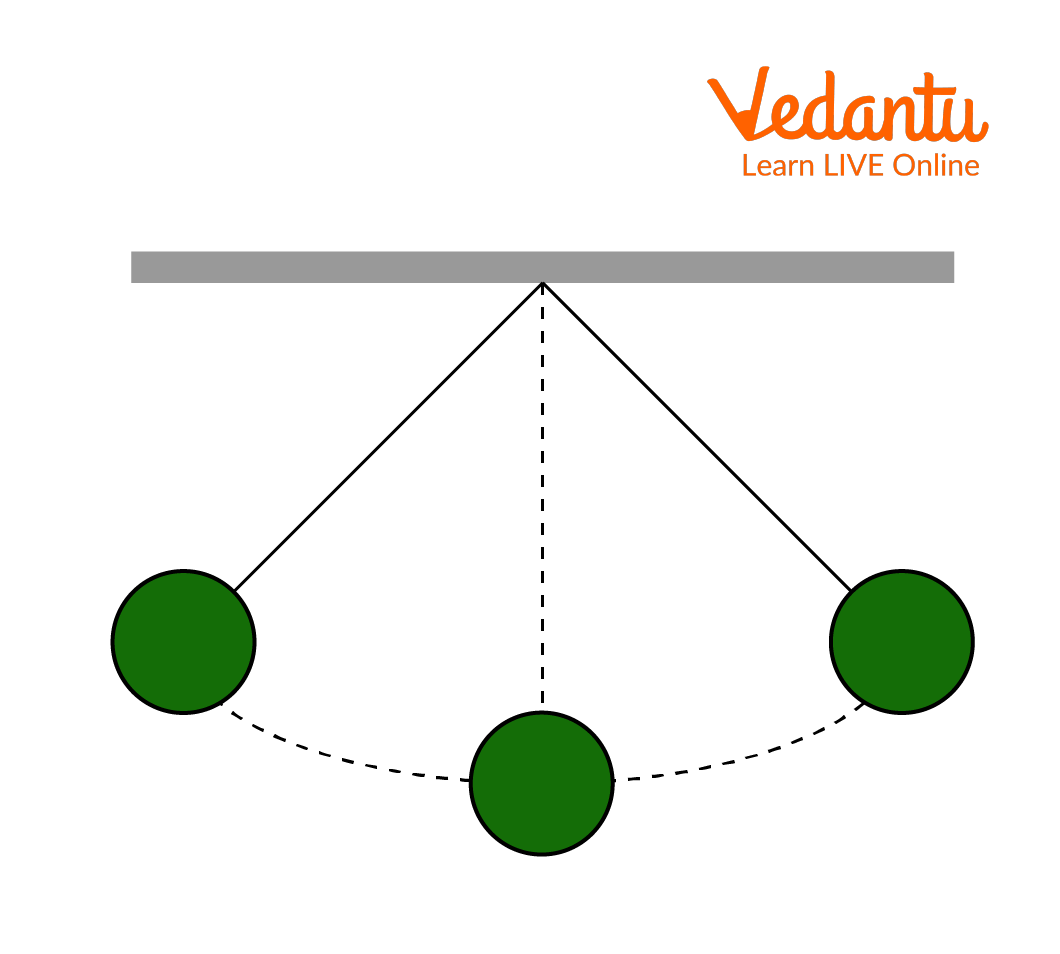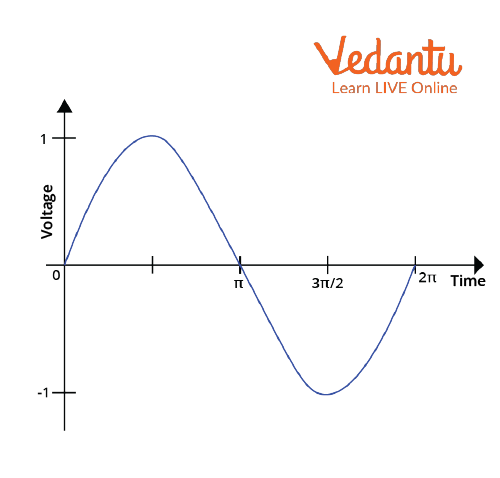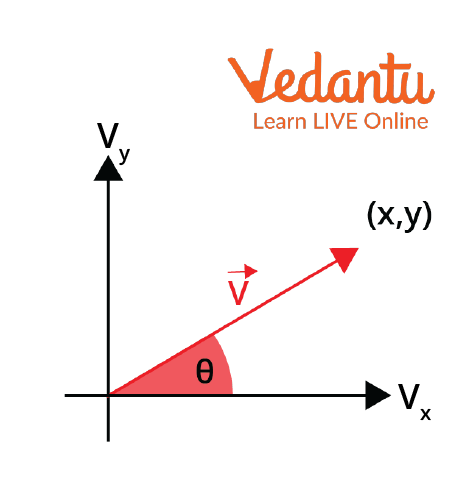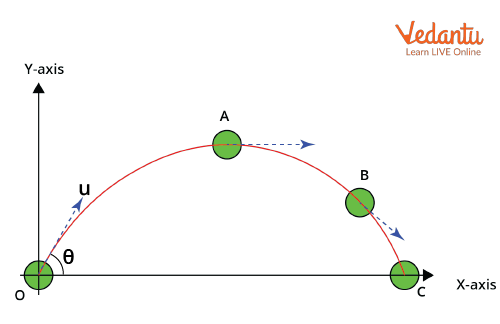Courses
Courses for Kids
Free study material
Free LIVE classes
More

# Motion in 2D and Its Dimensions for JEELIVE
Join Vedantu’s FREE Mastercalss

## What is Motion in 2D?

Motion is the change of position of an object or a body. It could be a one-dimensional, two-dimensional, or three-dimensional motion. The motion of an object or particle in a plane is referred to as two-dimensional motion. Motion in two dimensions can further be divided into circular, projectile, and planar motion. Their examples include: a boy throwing a ball towards his friend is an example of projectile motion; rotating ceiling fan is an example of circular motion. Now, how do we simplify motion in two dimensions? We divide it into one dimension, correlating the equations and then calculating the unknowns.

## What is Motion?

The rate of change of position is known as motion. It can be different physical quantities like displacement, acceleration, velocity, speed, and so on. A car accelerating, an apple falling from a tree, water gushing out of a tap, etc. are all examples of motion. Every physical process around us is in some kind of fast or slow motion. Take, for example, distance. It is the change in position of an object with respect to time. Acceleration, on the other hand, is the change in velocity of an object with respect to time. Be it velocity, distance, acceleration, or displacement, all are used to describe motion.

## What are the Types of Motion?

Depending on the type of motion an object goes through, motion can be classified into different types, which include:

• Linear motion of objects or rectilinear motion

• Rotational Motion of an object

• Oscillatory Motion

The rotation of a car wheel, a pendulum swinging to and fro, a ball rolling from an inclined surface, a car accelerating at a constant speed or variable speeds are all examples of the different types of motion listed above. Let us further understand this with a few more examples.

## Explanation of the Types of Motion with Examples

### Linear or Rectilinear Motion

As the name suggests, a linear or rectilinear motion is the motion of an object in a straight line. We witness several examples of linear motion in our day-to-day lives. Some of these include the following:

1. A Sliding Door

A sliding door is a perfect example of linear motion. The to-and-fro motion of the sliding door allows a person to enter or close the door.

2. A Person firing a Bullet

A person firing a bullet is another good example of linear motion. When the bullet is fired, it leaves the barrel in a straight line.Firing a Bullet

3. The Motion of a Car on a flat Road

The forward or backward motion of a car on a flat road is another great example to exhibit linear motion.

## Rotational Motion

Rotational or circular motion is the motion of a rigid body moving with a constant angular velocity about an axis. The rotational motion does not change the shape or size of an object. Let us further understand this with a few examples.

1. Earth Rotating about its Axis

The rotation of the Earth around its axis is a perfect example of rotational motion. The Earth rotates at a very slow and constant speed about its axis.

2. Rotation of a Ceiling Fan

A ceiling fan mounted on the roof of your house rotates in a clockwise direction when it is switched on. The speed of the fan at a time remains the same until it is changed using a regulator.

3. Rotation of the Minute or Hour Hand of the Clock

The rotation of different hands of the clock is another example of rotational motion. The hands of the clock move in a clockwise direction to complete a whole rotation around a fixed point.

## Oscillatory Motion

Oscillatory motion is also called periodic or repetitive motion. It is the to-and-fro motion of an object from its mean position. Some examples of oscillatory motion include the following.

1. A Pendulum Swinging

The to-and-fro motion of a pendulum from its mean position is an example of oscillatory motion.Pendulum Swinging

2. Alternating Current

The AC supply that we get in our households is another example of an oscillatory motion as current reverses its direction at regular intervals.Alternating Current or AC

3. Vibrating string of a guitar or any musical instrument

The strings of the guitar vibrate when a person plucks them using a pick. It is another good example of an oscillatory motion.

## What are the Dimensions of Acceleration?

Acceleration is the rate of change of velocity. To denote the dimensions of acceleration, they are denoted in terms of mass, length, and time. Let us derive this.

Let

Mass = M

Length = L

Time = T

Acceleration = a

Velocity = v

Now the dimensional formula for displacement, time, and velocity are:

Displacement = M0L1T0

Time=M0L0T1

We know, velocity=displacement $\times$ time-1

=(M0L1T0)$\times$(M0L0T1)-1

=(M0L1T0)$\times$(M0L1T-1)

Velocity=M0L1T-1

Now, we know acceleration=velocity $\times$time-1

=(M0L1T-1)$\times$(M0L0T1)

=M0L1T-2

Thus, the dimensions of acceleration are M0L1T-2.

Now that we have discussed types of motion with examples, we will look at some of the solved examples related to the same concept.

## Visualisation of Vector in 2D

2D objects or two-dimensional objects are nothing but the objects that have a length and a breadth. For a vector to be denoted in 2D, its components are represented on the a-axis and y-axis, respectively.  Mathematically, these will be denoted as VX and VY. $\theta$ is the angle that the vector $\vec V$ with the x-axis.2D Vector

## Relative Velocity

Relative velocity is the velocity of an object with another object. To understand how two objects are moving with respect to another, we use the concept of relative velocity. The two objects in this case might move in the same direction or in the opposite direction. Suppose that one object has velocity v1 and another has velocity v2, then the relative velocity of one with respect to the second is

\begin{align}&\vec{V}_{12}=\vec{V}_{2}-\vec{V}_{1}\\&\vec{V}_{12}=\vec{V}_{2}+\vec{V}_{1} \end{align}

First expression is valid when both objects have velocities in opposite directions and the second is valid when both objects have velocities in the same direction.

## Projectile Motion

The motion of an object in a curved path with constant acceleration is known as projectile motion. The motion in this case is along the x-axis and the y-axis.Projectile Motion

The acceleration component along the x-axis is 0, whereas in the vertical direction it is -g. However, the component of velocity along the x-axis is  $u \cos (\theta)$ and $u \sin (\theta)$, respectively.

The equation of a projectile or the trajectory formula as it is known is given as

$y=x \tan \theta-\dfrac{g x^{2}}{2 u^{2} \cos ^{2} \theta}$

Here, x and y are the horizontal and vertical components, respectively, and u is the initial velocity and g is the acceleration due to gravity. There are other important equations related to the projectile motion which include the time of flight (T), maximum height (H), and the horizontal range of the projectile (R). These equations are

\begin{align} &T=\dfrac{2 u \sin \theta}{g} \\ &H=\dfrac{u^{2} \sin ^{2} \theta}{2 g} \\ &R=\dfrac{u^{2} \sin 2 \theta}{g} \end{align}

Here, u is the initial velocity, g is the acceleration due to gravity, and θ at which the projectile was thrown with respect to the horizontal.

These are the equations of motion of the projectile.

## Equation of Motions in Two Dimensions

The three equations of motion in two dimensions x and y are given as:

\begin{align} &v_{x}=u_{x 0}+a_{x} t \\ &\Delta x=u_{x 0} t+\dfrac{1}{2} a_{x} t^{2} \\ &v_{x}^{2}-u_{x 0}^{2}=2 a_{x} \Delta x \end{align}

Similarly, the equations can be written in y directions.

\begin{align} &v_{y}=u_{y 0}+a_{y} t \\ &\Delta y=u_{y 0} t+\dfrac{1}{2} a_{y} t^{2} \\ &v_{y}^{2}-u_{y 0}^{2}=2 a_{y} \Delta y \end{align}

## Solved Examples for Two-Dimensional Motion

Example 1: A particle is moving with an initial velocity (2i + 4j) $\dfrac{m}{s}$ and has constant acceleration of  (10i + 2j) $\dfrac{m}{s^2}$. Calculate the final velocity and displacement after 6 seconds.

Solution: The above problem can be solved by dividing velocity and acceleration into one dimension. Thus, along the x-axis, we have initial velocity as 2 $\dfrac{m}{s}$, acceleration as 4 $\dfrac{m}{s^2}$. Using the first equation of motion, we have v=u+at. Substituting the values above in this, we get

\begin{align} &v=2+10 \times 6 \\ &v=62 m s^{-1} \end{align}

Now, using the second equation of motion, we have $s=u t+\dfrac{1}{2} a t^{2}$. Substituting the above values we get,

\begin{align} &s=2 \times 6+\dfrac{1}{2}(10) \times 6^{2} \\ &s=12+180 \\ &s=192 \mathrm{~m} \end{align}

Now, we will calculate the same along the y-direction.

We get the value of velocity as

\begin{align} &v=4+2 \times 6 \\ &v=16 \mathrm{~ms}^{-1} \end{align}

Similarly, the displacement will be

\begin{align} &s=4 \times 6+\dfrac{1}{2} \times 2 \times 6^{2} \\ &s=24+36 \\ &s=60 \mathrm{~m} \end{align}

Thus, final velocity = $v=v_{x} \hat{i}+v_{y} \hat{j}=62 \hat{i}+16 \hat{j}$

or

\begin{align} v &=\sqrt{62^{2}+16^{2}} \\ =v &=64 ms^{-1} \end{align}

The final displacement is

\begin{align} &s=s_{x} \hat{i}+s_{y} \hat{j} \\ &s=192 \hat{i}+60 \hat{j} \\ &\text { or } \\ &s=\sqrt{192^{2}+60^{2}} \\ &s=201.15 \mathrm{~m} \end{align}

Hence, the final velocity and displacement after 6 seconds are $62 \hat{i}+16 \hat{j}$ and $201.15 \mathrm{~m}$.

Example 2: An object is moving in a plane with a velocity $v=4 t \hat{i}+6 t^{3} \hat{j}$. Find the acceleration of the object between time intervals (0,2).

Solution: Acceleration is denoted as $a=\dfrac{\Delta v}{\Delta t}$

At 0 time interval, velocity will be zero, whereas at t=2 seconds, velocity will be

\begin{align} &v=4 \times 2 \hat{i}+6 \times 2^{3} \hat{j} \\ &v=8 \hat{i}+48 \hat{j} \end{align}

Therefore, acceleration will be

\begin{align} &\Rightarrow \dfrac{8 \hat{i}+48 \hat{j}}{2} \\ &\Rightarrow 4 \hat{i}+24 \hat{j} \end{align}

Hence, the acceleration of the object between time intervals (0,2) is $4 \hat{i}+24 \hat{j}$.

## Conclusion

Two-dimensional motion is an important concept that is used to describe displacement, acceleration, speed, etc. It is the change in motion of an object with time. In our day-to-day life, we experience different kinds of motion, be it one-dimensional, two-dimensional, or even three-dimensional. The difference of an object gives rise to the concepts of the frame of reference and relative motion. These are extremely fundamental concepts when dealing with two-dimensional motion problems.

Last updated date: 15th Sep 2023
Total views: 127.2k
Views today: 3.27k

## FAQs on Motion in 2D and Its Dimensions for JEE

1. What is a frame of reference?

A frame of reference is an important concept in Physics. It is especially helpful when we are trying to calculate a value based on set values or criteria. It usually consists of a coordinate system or setting up coordinates in relation to the motion of an observer.

For example, if a person is looking at a moving train, and another person sitting inside the train is waving his hand towards him. In such a case, we take two frames of reference, one of the person standing at the platform watching the moving train, and the other one is of the person sitting inside the train. To the person standing on the platform, the person sitting inside the train seems to be moving. But from his/her frame of reference, the person sitting inside is at rest.

2. What is relative motion?

The motion of one body with respect to another, which could be at rest or in motion, is known as relative motion. The concept of a frame of reference was introduced to understand relative motion in-depth. The motion in case of a relative motion depends on the frame of reference or location of the observer. Motion can also be viewed from a fixed point of view. This type of motion is known as absolute motion.